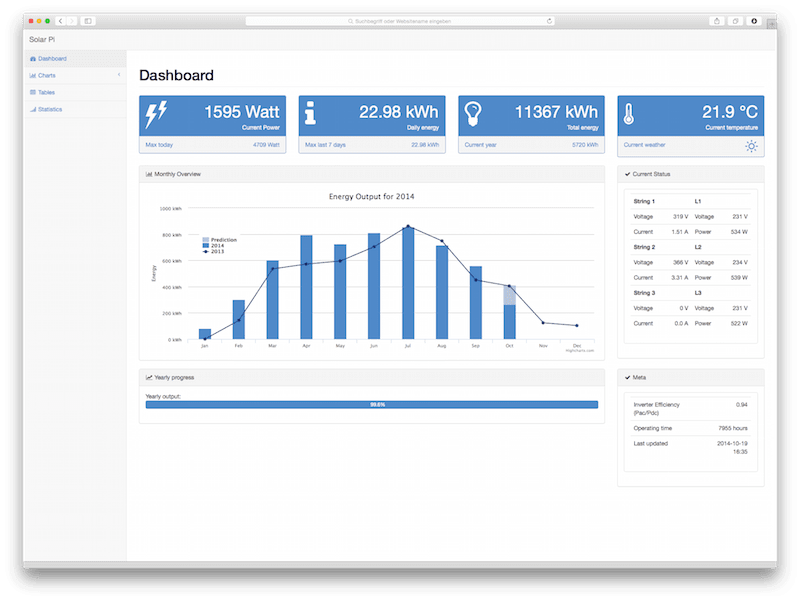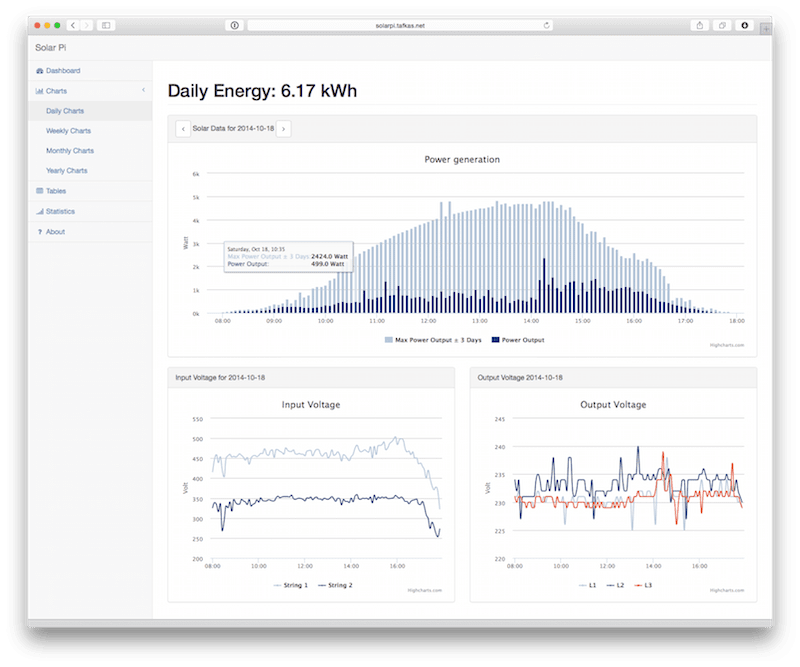After collecting some photovoltaic data using PikoPy and a some readings from the residential meter it was time to put everything together. The data is collected by a couple of scripts triggered by a cronjob every five minutes.

``````\$ crontab -l

*/5 * * * * python /home/solarpi/kostal_piko.py
*/5 * * * * python /home/solarpi/collect_meter.py
*/15 * * * * python /home/solarpi/collect_weather.py
``````

The results are then written into a SQLite database. I chose SQLite over PostgreSQL for its lighter footprint on the Raspberry Pi. And since we will have no concurrent writes SQLite should be capable serving as a database backend.

The sole purpose of the web application is to fetch data from the database and render the results. I decided to use Flask, a microframework for Python based on Werkzeug and Jinja 2. For the Frontend I used the Bootstrap based SB Admin 2 Theme.

Initially I used SQLAlchemy as an Object Relational Mapper where I defined my models in Python:

``````class PVData(SurrogatePK, Model):

__tablename__ = 'pv_data'
id = Column(db.Integer(), nullable=False, primary_key=True)
created_at = Column(db.Text(), nullable=False, default=dt.datetime.utcnow)
dc_1_u = Column(db.Integer(), nullable=True)
dc_1_i = Column(db.Float(), nullable=True)
ac_1_u = Column(db.Integer(), nullable=True)
ac_1_p = Column(db.Integer(), nullable=True)
dc_2_u = Column(db.Integer(), nullable=True)
.
.
.
current_power = Column(db.Integer(), nullable=True)
daily_energy = Column(db.Float(), nullable=True)
total_energy = Column(db.Integer(), nullable=True)
``````

While using an ORM is very helpful for CRUD operation I decided to keep my read queries using raw SQL instead.

The main view is a dashboard presenting all kinds of interesting KPIs like yielded, imported, exported Energy and how much money was received for the sold energy:Furthermore there are detailed charts available for each day at a five minute interval, or the last 7 days and 30 days, respectively:The project is live at: http://solarpi.tafkas.net

The code is on Github at https://github.com/Tafkas/solarpi